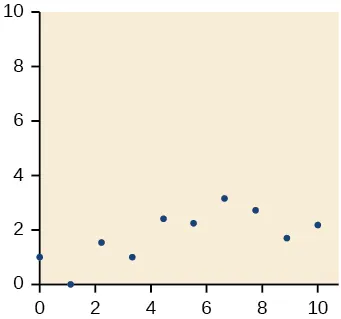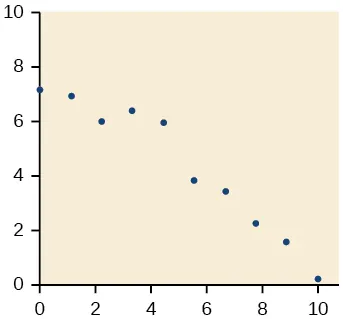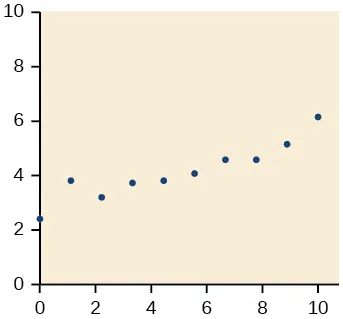Precalculus

# 2.4Fitting Linear Models to Data

Precalculus2.4 Fitting Linear Models to Data

### Learning Objectives

In this section, you will:

• Draw and interpret scatter plots.
• Find the line of best fit.
• Distinguish between linear and nonlinear relations.
• Use a linear model to make predictions.

A professor is attempting to identify trends among final exam scores. His class has a mixture of students, so he wonders if there is any relationship between age and final exam scores. One way for him to analyze the scores is by creating a diagram that relates the age of each student to the exam score received. In this section, we will examine one such diagram known as a scatter plot.

### Drawing and Interpreting Scatter Plots

A scatter plot is a graph of plotted points that may show a relationship between two sets of data. If the relationship is from a linear model, or a model that is nearly linear, the professor can draw conclusions using his knowledge of linear functions. Figure 1 shows a sample scatter plot.

Figure 1 A scatter plot of age and final exam score variables

Notice this scatter plot does not indicate a linear relationship. The points do not appear to follow a trend. In other words, there does not appear to be a relationship between the age of the student and the score on the final exam.

### Example 1

#### Using a Scatter Plot to Investigate Cricket Chirps

Table 1 shows the number of cricket chirps in 15 seconds, for several different air temperatures, in degrees Fahrenheit5. Plot this data, and determine whether the data appears to be linearly related.

 Chirps 44 35 20.4 33 31 35 18.5 37 26 Temperature 80.5 70.5 57 66 68 72 52 73.5 53
Table 1

### Finding the Line of Best Fit

Once we recognize a need for a linear function to model that data, the natural follow-up question is “what is that linear function?” One way to approximate our linear function is to sketch the line that seems to best fit the data. Then we can extend the line until we can verify the y-intercept. We can approximate the slope of the line by extending it until we can estimate the $riserun.riserun.$

### Example 2

#### Finding a Line of Best Fit

Find a linear function that fits the data in Table 1 by “eyeballing” a line that seems to fit.

#### Analysis

This linear equation can then be used to approximate answers to various questions we might ask about the trend.

#### Recognizing Interpolation or Extrapolation

While the data for most examples does not fall perfectly on the line, the equation is our best guess as to how the relationship will behave outside of the values for which we have data. We use a process known as interpolation when we predict a value inside the domain and range of the data. The process of extrapolation is used when we predict a value outside the domain and range of the data.

Figure 4 compares the two processes for the cricket-chirp data addressed in Example 2. We can see that interpolation would occur if we used our model to predict temperature when the values for chirps are between 18.5 and 44. Extrapolation would occur if we used our model to predict temperature when the values for chirps are less than 18.5 or greater than 44.

Figure 4 Interpolation occurs within the domain and range of the provided data whereas extrapolation occurs outside.

There is a difference between making predictions inside the domain and range of values for which we have data and outside that domain and range. Predicting a value outside of the domain and range has its limitations. When our model no longer applies after a certain point, it is sometimes called model breakdown. For example, predicting a cost function for a period of two years may involve examining the data where the input is the time in years and the output is the cost. But if we try to extrapolate a cost when $x=50,x=50,$ that is in 50 years, the model would not apply because we could not account for factors fifty years in the future.

### Interpolation and Extrapolation

Different methods of making predictions are used to analyze data.

• The method of interpolation involves predicting a value inside the domain and/or range of the data.
• The method of extrapolation involves predicting a value outside the domain and/or range of the data.
• Model breakdown occurs at the point when the model no longer applies.

### Example 3

#### Understanding Interpolation and Extrapolation

Use the cricket data from Table 1 to answer the following questions:

1. Would predicting the temperature when crickets are chirping 30 times in 15 seconds be interpolation or extrapolation? Make the prediction, and discuss whether it is reasonable.
2. Would predicting the number of chirps crickets will make at 40 degrees be interpolation or extrapolation? Make the prediction, and discuss whether it is reasonable.

#### Analysis

Our model predicts the crickets would chirp 8.33 times in 15 seconds. While this might be possible, we have no reason to believe our model is valid outside the domain and range. In fact, generally crickets stop chirping altogether below around 50 degrees.

### Try It #1

According to the data from Table 1, what temperature can we predict it is if we counted 20 chirps in 15 seconds?

#### Finding the Line of Best Fit Using a Graphing Utility

While eyeballing a line works reasonably well, there are statistical techniques for fitting a line to data that minimize the differences between the line and data values6. One such technique is called least squares regression and can be computed by many graphing calculators, spreadsheet software, statistical software, and many web-based calculators7. Least squares regression is one means to determine the line that best fits the data, and here we will refer to this method as linear regression.

### How To

Given data of input and corresponding outputs from a linear function, find the best fit line using linear regression.

1. Enter the input in List 1 (L1).
2. Enter the output in List 2 (L2).
3. On a graphing utility, select Linear Regression (LinReg).

### Example 4

#### Finding a Least Squares Regression Line

Find the least squares regression line using the cricket-chirp data in Table 1.

#### Analysis

Notice that this line is quite similar to the equation we “eyeballed” but should fit the data better. Notice also that using this equation would change our prediction for the temperature when hearing 30 chirps in 15 seconds from 66 degrees to:

The graph of the scatter plot with the least squares regression line is shown in Figure 6.

Figure 6

### Q&A

Will there ever be a case where two different lines will serve as the best fit for the data?

No. There is only one best fit line.

### Distinguishing Between Linear and Non-Linear Models

As we saw above with the cricket-chirp model, some data exhibit strong linear trends, but other data, like the final exam scores plotted by age, are clearly nonlinear. Most calculators and computer software can also provide us with the correlation coefficient, which is a measure of how closely the line fits the data. Many graphing calculators require the user to turn a ”diagnostic on” selection to find the correlation coefficient, which mathematicians label as $r.r.$ The correlation coefficient provides an easy way to get an idea of how close to a line the data falls.

We should compute the correlation coefficient only for data that follows a linear pattern or to determine the degree to which a data set is linear. If the data exhibits a nonlinear pattern, the correlation coefficient for a linear regression is meaningless. To get a sense for the relationship between the value of $rr$ and the graph of the data, Figure 7 shows some large data sets with their correlation coefficients. Remember, for all plots, the horizontal axis shows the input and the vertical axis shows the output.

Figure 7 Plotted data and related correlation coefficients. (credit: “DenisBoigelot,” Wikimedia Commons)

### Correlation Coefficient

The correlation coefficient is a value, $r,r,$ between –1 and 1.

• r > 0 suggests a positive (increasing) relationship
• r < 0 suggests a negative (decreasing) relationship
• The closer the value is to 0, the more scattered the data.
• The closer the value is to 1 or –1, the less scattered the data is.

### Example 5

#### Finding a Correlation Coefficient

Calculate the correlation coefficient for cricket-chirp data in Table 1.

### Predicting with a Regression Line

Once we determine that a set of data is linear using the correlation coefficient, we can use the regression line to make predictions. As we learned above, a regression line is a line that is closest to the data in the scatter plot, which means that only one such line is a best fit for the data.

### Example 6

#### Using a Regression Line to Make Predictions

Gasoline consumption in the United States has been steadily increasing. Consumption data from 1994 to 2004 is shown in Table 38. Determine whether the trend is linear, and if so, find a model for the data. Use the model to predict the consumption in 2008.

 Year '94 '95 '96 '97 '98 '99 '00 '01 '02 '03 '04 Consumption (billions of gallons) 113 116 118 119 123 125 126 128 131 133 136
Table 3

The scatter plot of the data, including the least squares regression line, is shown in Figure 8.

Figure 8

### Try It #2

Use the model we created using technology in Example 6 to predict the gas consumption in 2011. Is this an interpolation or an extrapolation?

Access these online resources for additional instruction and practice with fitting linear models to data.

### 2.4 Section Exercises

#### Verbal

1.

Describe what it means if there is a model breakdown when using a linear model.

2.

What is interpolation when using a linear model?

3.

What is extrapolation when using a linear model?

4.

Explain the difference between a positive and a negative correlation coefficient.

5.

Explain how to interpret the absolute value of a correlation coefficient.

#### Algebraic

6.

A regression was run to determine whether there is a relationship between hours of TV watched per day $(x)(x)$ and number of sit-ups a person can do $(y).(y).$ The results of the regression are given below. Use this to predict the number of sit-ups a person who watches 11 hours of TV can do.

$y=ax+b a=−1.341 b=32.234 r=−0.896 y=ax+b a=−1.341 b=32.234 r=−0.896$
7.

A regression was run to determine whether there is a relationship between the diameter of a tree ( $x,x,$ in inches) and the tree’s age ( $y,y,$ in years). The results of the regression are given below. Use this to predict the age of a tree with diameter 10 inches.

$y=ax+b a=6.301 b=−1.044 r=−0.970 y=ax+b a=6.301 b=−1.044 r=−0.970$

For the following exercises, draw a scatter plot for the data provided. Does the data appear to be linearly related?

8.
 0 2 4 6 8 10 –22 –19 –15 –11 –6 –2
9.
 1 2 3 4 5 6 46 50 59 75 100 136
10.
 100 250 300 450 600 750 12 12.6 13.1 14 14.5 15.2
11.
 1 3 5 7 9 11 1 9 28 65 125 216
12.

For the following data, draw a scatter plot. If we wanted to know when the population would reach 15,000, would the answer involve interpolation or extrapolation? Eyeball the line, and estimate the answer.

YearPopulation
199011,500
199512,100
200012,700
200513,000
201013,750
13.

For the following data, draw a scatter plot. If we wanted to know when the temperature would reach 28 °F, would the answer involve interpolation or extrapolation? Eyeball the line and estimate the answer.

 Temperature, °F 16 18 20 25 30 Time, seconds 46 50 54 55 62

#### Graphical

For the following exercises, match each scatterplot with one of the four specified correlations in Figure 9 and Figure 10.

Figure 9
Figure 10
14.

$r=0.95r=0.95$

15.

$r=−0.89r=−0.89$

16.

$r=0.26r=0.26$

17.

$r=−0.39r=−0.39$

For the following exercises, draw a best-fit line for the plotted data.

18.19.20.21.#### Numeric

22.

The U.S. Census tracks the percentage of persons 25 years or older who are college graduates. That data for several years is given in Table 49. Determine whether the trend appears linear. If so, and assuming the trend continues, in what year will the percentage exceed 35%?

199021.3
199221.4
199422.2
199623.6
199824.4
200025.6
200226.7
200427.7
200628
200829.4
Table 4
23.

The U.S. import of wine (in hectoliters) for several years is given in Table 5. Determine whether the trend appears linear. If so, and assuming the trend continues, in what year will imports exceed 12,000 hectoliters?

YearImports
19922665
19942688
19963565
19984129
20004584
20025655
20046549
20067950
20088487
20099462
Table 5
24.

Table 6 shows the year and the number of people unemployed in a particular city for several years. Determine whether the trend appears linear. If so, and assuming the trend continues, in what year will the number of unemployed reach 5?

YearNumber Unemployed
1990750
1992670
1994650
1996605
1998550
2000510
2002460
2004420
2006380
2008320
Table 6

#### Technology

For the following exercises, use each set of data to calculate the regression line using a calculator or other technology tool, and determine the correlation coefficient to 3 decimal places of accuracy.

25.
 $xx$ 8 15 26 31 56 $yy$ 23 41 53 72 103
26.
 $xx$ 5 7 10 12 15 $yy$ 4 12 17 22 24
27.
 $xx$ $yy$ $xx$ $y y$ 3 21.9 11 15.76 4 22.22 12 13.68 5 22.74 13 14.1 6 22.26 14 14.02 7 20.78 15 11.94 8 17.6 16 12.76 9 16.52 17 11.28 10 18.54 18 9.1
28.
 $xx$ $yy$ 4 44.8 5 43.1 6 38.8 7 39 8 38 9 32.7 10 30.1 11 29.3 12 27 13 25.8
29.
 $xx$ 21 25 30 31 40 50 $yy$ 17 11 2 -1 -18 -40
30.
 $xx$ 100 80 60 55 40 20 $yy$ 2000 1798 1589 1580 1390 1202
31.
 $xx$ 900 988 1000 1010 1200 1205 $yy$ 70 80 82 84 105 108

#### Extensions

32.

Graph $f(x)=0.5x+10f(x)=0.5x+10$. Pick a set of 5 ordered pairs using inputs and use linear regression to verify that the function is a good fit for the data.

33.

Graph $f(x)=−2x−10f(x)=−2x−10$. Pick a set of 5 ordered pairs using inputs and use linear regression to verify the function.

For the following exercises, consider this scenario: The profit of a company decreased steadily over a ten-year span. The following ordered pairs shows dollars and the number of units sold in hundreds and the profit in thousands of over the ten-year span, (number of units sold, profit) for specific recorded years:

.

34.

Use linear regression to determine a function $PP$ where the profit in thousands of dollars depends on the number of units sold in hundreds.

35.

Find to the nearest tenth and interpret the x-intercept.

36.

Find to the nearest tenth and interpret the y-intercept.

#### Real-World Applications

For the following exercises, consider this scenario: The population of a city increased steadily over a ten-year span. The following ordered pairs shows the population and the year over the ten-year span, (population, year) for specific recorded years:

37.

Use linear regression to determine a function $y,y,$ where the year depends on the population. Round to three decimal places of accuracy.

38.

Predict when the population will hit 8,000.

For the following exercises, consider this scenario: The profit of a company increased steadily over a ten-year span. The following ordered pairs show the number of units sold in hundreds and the profit in thousands of over the ten year span, (number of units sold, profit) for specific recorded years:

.

39.

Use linear regression to determine a function y, where the profit in thousands of dollars depends on the number of units sold in hundreds .

40.

Predict when the profit will exceed one million dollars.

For the following exercises, consider this scenario: The profit of a company decreased steadily over a ten-year span. The following ordered pairs show dollars and the number of units sold in hundreds and the profit in thousands of over the ten-year span (number of units sold, profit) for specific recorded years:

41.

Use linear regression to determine a function y, where the profit in thousands of dollars depends on the number of units sold in hundreds .

42.

Predict when the profit will dip below the \$25,000 threshold.

### Footnotes

• 5Selected data from http://classic.globe.gov/fsl/scientistsblog/2007/10/. Retrieved Aug 3, 2010
• 6Technically, the method minimizes the sum of the squared differences in the vertical direction between the line and the data values.
• 7For example, http://www.shodor.org/unchem/math/lls/leastsq.html
• 8http://www.bts.gov/publications/national_transportation_statistics/2005/html/table_04_10.html
• 9http://www.census.gov/hhes/socdemo/education/data/cps/historical/index.html. Accessed 5/1/2014.
Order a print copy

As an Amazon Associate we earn from qualifying purchases.